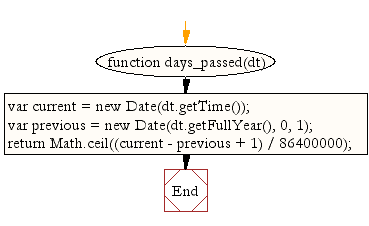# JavaScript: Count the number of days passed since beginning of the year

## JavaScript Datetime: Exercise-16 with Solution

Write a JavaScript function to count the number of days passed since beginning of the year.

Test Data:
console.log(days_passed(new Date(2015, 0, 15)));
15
console.log(days_passed(new Date(2015, 11, 14)));
348

Sample Solution:-

HTML Code:

``````<!DOCTYPE html>
<html>
<head>
<meta charset="utf-8">
<title>JavaScript function to count the number of days passed since beginning of the year</title>
</head>
<body>

</body>
</html>
```
```

JavaScript Code:

``````function days_passed(dt) {
var current = new Date(dt.getTime());
var previous = new Date(dt.getFullYear(), 0, 1);

return Math.ceil((current - previous + 1) / 86400000);
}
console.log(days_passed(new Date(2015, 0, 15)));
console.log(days_passed(new Date(2015, 11, 14)));
```
```

Sample Output:

```15
348
```

Flowchart:Live Demo:

See the Pen JavaScript - Count the number of days passed since beginning of the year-date-ex- 16 by w3resource (@w3resource) on CodePen.

Improve this sample solution and post your code through Disqus

What is the difficulty level of this exercise?

﻿

## JavaScript: Tips of the Day

Converts the given array elements into <li> tags and appends them to the list of the given id.

Example:

```const tips_arrayToHtmlList = (arr, listID) =>(el => ((el = document.querySelector('#' + listID)),(el += arr.map(item => '<li>\${item}</li>').join(''))))();
console.log(tips_arrayToHtmlList(['item 1', 'item 2'], 'myListID'));
```

Output:

```"null\${item}\${item}"
```